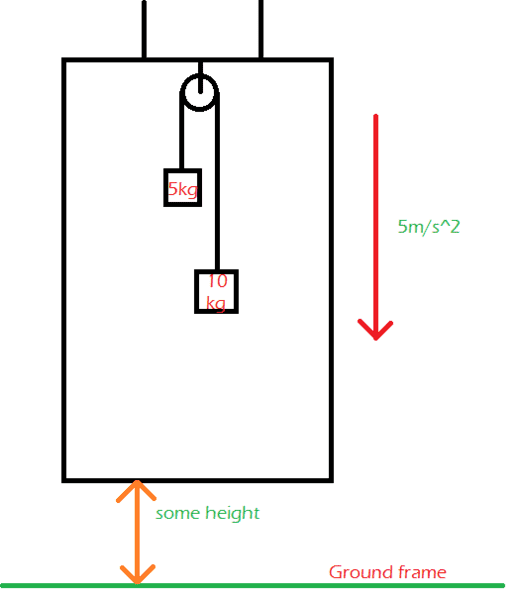# What will be the acceleration of a 10kg block w.r.t. the ground frame?

prakhargupta3301

## Homework Statement[/B]We have to find acceleration produced by 10 kg block on 5 kg block with respect to the ground.
Downward acceleration of life is 5m/s-2

f=ma
g=10m/s

## The Attempt at a Solution

Effective force at play on 10 kg block: mg- ma
= 100N-50N
= 50 Newtons
Effective force at play on 5 kg block: mg-ma
= (50-25)N
= 25 Newtons
The 10 kg block outmatched its nemesis (5kg block) by +25 Newtons. It's the heavier one.
25N=10kg*a
2.5m/s2

But this isn't the correct answer. The correct is 5 m/s2.

## Answers and Replies

Homework Helper
If the 10kg mass had fallen as was resting on the elevator floor, or the pulley jammed and the mass was hanging from the ceiling, then its acceleration w.r.t. ground would be ##5m/s^2##, as would be the entire elevator. Otherwise, it will be accelerating downward at a rate greater than ##5m/s^2##.

•haruspex and prakhargupta3301
prakhargupta3301
Otherwise, it will be accelerating downward at a rate greater than ##5m/s^2##.
Will it be 15m/s2?
Or 12.5 m/s2?
Or something else?

Homework Helper
No free answers. Do the math.
That said, I would work out the acceleration w.r.t. the elevator. Then add in the elevator acceleration.

prakhargupta3301
I did the math. But with respect to keeping the elevator as my reference and got 5m/s2 as acceleration of the system of the pulley in downward direction.
What I need help is with knowing, whether the acceleration would seem different from the inertial frame?
i.e would it still be 5m/s2, or 2.5m/s2 as I have already found?
Thank you.

Homework Helper
What I need help is with knowing, whether the acceleration would seem different from the inertial frame?
i.e would it still be 5m/s2, or 2.5m/s2 as I have already found?
Thank you.
The apparent "G force" in the elevator will be ##G-5m/s^2## or ##5m/s^2##.
If the 10kg mass was entirely free, it would fall ##5m/s^2## w.r.t. to the elevator and ##10m/s^2## w.r.t. the ground.
Attaching it to the 5kg mass through the pulley system will only slow it down - so the correct answer will be less than ##10m/s^2##.

Homework Helper
Gold Member
Effective force at play on 10 kg block: mg- ma
= 100N-50N
You seem to have assumed, wrongly, that the acceleration of the 10kg block inthe ground frame is 5m/s2.
Create unknowns for the tension in the cable and the acceleration of the 10kg block (either in the ground frame or relative to the lift - your choice).
Write the ΣF=ma equation for each block.
Downward acceleration of life is 5m/s-2
It can seem like that some days.
The correct is 5 m/s2.
It isn't that either.